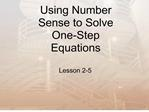# Using Number Sense to Solve One-Step Equations - PowerPoint PPT PresentationDownload PresentationUsing Number Sense to Solve One-Step Equations

Using Number Sense to Solve One-Step Equations
Download Presentation## Using Number Sense to Solve One-Step Equations

- - - - - - - - - - - - - - - - - - - - - - - - - - - E N D - - - - - - - - - - - - - - - - - - - - - - - - - - -
##### Presentation Transcript

1. Using Number Sense to Solve One-Step Equations Lesson 2-5

2. Vocabulary

3. Using Estimation Use mental math or estimating to determine the solutions to the equations. The same number properties apply to algebraic equations.

4. Properties

5. Example: 4x=32

6. Example: Estimate p � 4 = 7.97

7. Is the given number a solution to the equation?

8. Homework Time